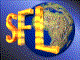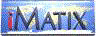| iMatix home page | << | < | > | >>SFLVersion 2.11

### strhash

```#include "sflstr.h"
qbyte
strhash (
const char *string)
```

#### Synopsis

Calculates a 32-bit hash value for the string. The string must end in a null. To use the result as a hash key, take the modulo over the hash table size. The algorithm was designed by Peter Weinberger. This version was adapted from Dr Dobb's Journal April 1996 page 26.

#### Examples

```    int index;
index = (int) strhash (name) % TABLE_SIZE;
```

#### Source Code - (sflstr.c)

```{
qbyte
high_bits,
hash_value = 0;

ASSERT (string);
while (*string)
{
hash_value = (hash_value << 4) + *string++;
if ((high_bits = hash_value & 0xF0000000L) != 0)
hash_value ^= high_bits >> 24;
hash_value &= ~high_bits;
}
return (hash_value);
}
```

 | << | < | > | >>Copyright © 1996-2000 iMatix Corporation